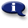# .

## Syllabus Information

Spring 2022
Dec 03,2023Use this page to maintain syllabus information, learning objectives, required materials, and technical requirements for the course.

Syllabus Information
MTH 065B - Elementary Algebra: Part B
Associated Term: Spring 2022
Learning Objectives: Upon successful completion of this course, the student will: 1- Maintain, use, and expand skills and concepts learned in previous mathematics courses. 2- Perform addition, subtraction, multiplication, and division of rational numbers. 3- Use and apply the concepts and language of algebraic expressions. 4- Solve linear equations and inequalities. 5- Interpret information represented numerically and graphically, and recognize the linear relationships represented verbally, numerically, graphically, and algebraically. 6- Evaluate and simplify expressions using the rules of exponents. 7- Use scientific notation. 8- Use the terminology of polynomials and perform algebraic operations with polynomials. 9- Recognize and use the terminology of polynomials. 10- Evaluate polynomials. 11- Add, subtract, and multiply polynomials. 12- Divide a polynomial by a monomial. 13- Factor polynomials. 14- Factor polynomials by removing a common monomial factor. 15- Factor trinomials. 16- Factor special products. 17- Make appropriate and efficient use of a scientific calculator. (Note: Students will be expected to demonstrate achievement of some objectives without the use of a calculator.)
Required Materials:
Technical Requirements: